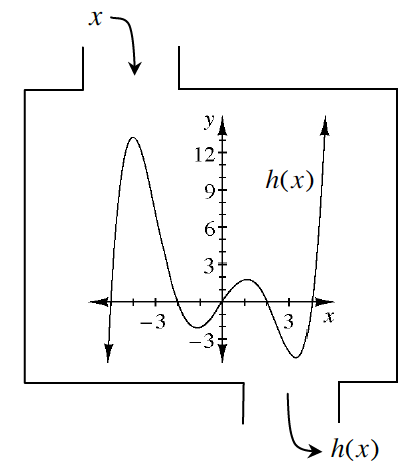### Home > CCAA8 > Chapter 2 Unit 2 > Lesson CCA: 2.1.1 > Problem2-8

2-8.

Examine the relation $h(x)$ defined at right. Then estimate the values below.

1.  $h(1)$

1.  $h(3)$

1.  $x$ when $h(x)=0$

1.  $h(−1)$

1.  $h(−4)$• Look at the graph to estimate the $y$-value for each of the given $x$-values.

There are 5 $x$-intercepts on the graph.

For part (c), you are being asked to find the values for $x$ when $y=0$. (Find the $x$-intercepts).

(a): $2$   (b): $−4$